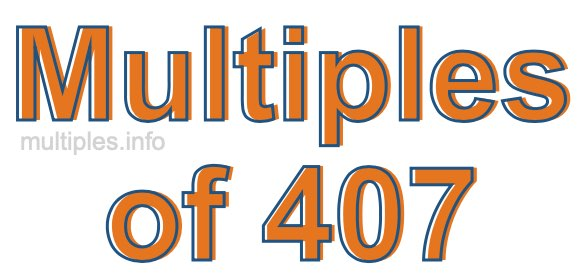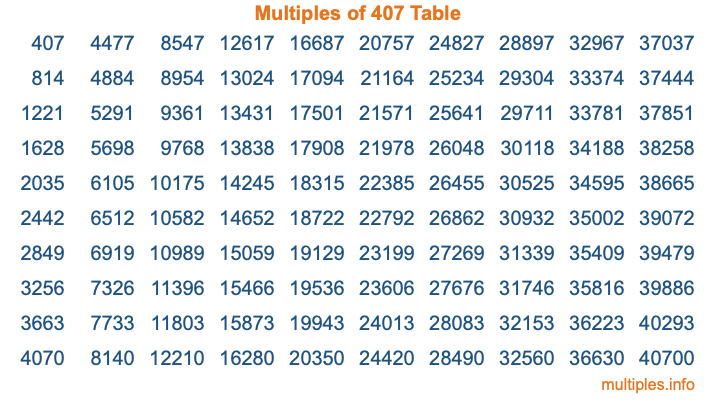Multiples of 407Welcome to the Multiples of 407 page. Here we will first teach you everything you will ever need to know about the multiples of 407, and then give you a study guide summary of everything we taught you to make sure you remember it all. Use this page to look up facts and learn information about the multiples of 407. This page will make you a multiples of four hundred seven expert!

Definition of Multiples of 407
Multiples of 407 are all the numbers that when divided by 407 equal an integer. Each of the multiples of 407 are called a multiple. A multiple of 407 is created by multiplying 407 by an integer.

Therefore, to create a list of multiples of 407, you start with 1 multiplied by 407, then 2 multiplied by 407, then 3 multiplied by 407, and so on for as long as you want. Thus, the list of the first five multiples of 407 is 407, 814, 1221, 1628, and 2035. To see a larger list of multiples of 407, see the printable image of Multiples of 407 further down on this page. We also have a category where you can choose any nth multiple of 407.

Multiples of 407 Checker
The Multiples of 407 Checker below checks to see if any number of your choice is a multiple of 407. In other words, it checks to see if there is any number (integer) that when multiplied by 407 will equal your number. To do that, we divide your number by 407. If the the quotient is an integer, then your number is a multiple of 407.

Is  a multiple of 407?

Least Common Multiple of 407 and ...
A Least Common Multiple (LCM) is the lowest multiple that two or more numbers have in common. This is also called the smallest common multiple or lowest common multiple and is useful to know when you are adding our subtracting fractions. Enter one or more numbers below (407 is already entered) to find the LCM.

Check out our LCM Calculator if you need more details about the Least Common Multiple or if you need the LCM for different numbers for adding and subtraction fractions.

nth Multiple of 407
As we stated above, 407 is the first multiple of 407, 814 is the second multiple of 407, 1221 is the third multiple of 407, and so on. Enter a number below to find the nth multiple of 407.

th multiple of 407

Multiples of 407 vs Factors of 407
407 is a multiple of 407 and a factor of 407, but that is where the similarities end. All postive multiples of 407 are 407 or greater than 407. All positive factors of 407 are 407 or less than 407.

Below is the beginning list of multiples of 407 and the factors of 407 so you can compare:

Multiples of 407: 407, 814, 1221, 1628, 2035, etc.

Factors of 407: 1, 11, 37, 407

As you can see, the multiples of 407 are all the numbers that you can divide by 407 to get a whole number. The factors of 407, on the other hand, are all the whole numbers that you can multiply by another whole number to get 407.

It's also interesting to note that if a number (x) is a factor of 407, then 407 will also be a multiple of that number (x).

Multiples of 407 vs Divisors of 407
The divisors of 407 are all the integers that 407 can be divided by evenly. Below is a list of the divisors of 407.

Divisors of 407: 1, 11, 37, 407

The interesting thing to note here is that if you take any multiple of 407 and divide it by a divisor of 407, you will see that the quotient is an integer.

Multiples of 407 Table
Below is an image of the first 100 multiples of 407 in a table. The table is in chronological order, column by column. The first column has the first ten multiples of 407, the second column has the next ten multiples of 407, and so on.The Multiples of 407 Table is also referred to as the 407 Times Table or Times Table of 407. You are welcome to print out our table for your studies.

Negative Multiples of 407
Although not often discussed or needed in math, it is worth mentioning that you can make a list of negative multiples of 407 by multiplying 407 by -1, then by -2, then by -3, and so on, to get the following list of negative multiples of 407:

-407, -814, -1221, -1628, -2035, etc.

Multiples of 407 Summary
Below is a summary of important Multiples of 407 facts that we have discussed on this page. To retain the knowledge on this page, we recommend that you read through the summary and explain to yourself or a study partner why they hold true.

There are an infinite number of multiples of 407.

A multiple of 407 divided by 407 will equal a whole number.

407 divided by a factor of 407 equals a divisor of 407.

The nth multiple of 407 is n times 407.

The largest factor of 407 is equal to the first positive multiple of 407.

407 is a multiple of every factor of 407.

407 is a multiple of 407.

A multiple of 407 divided by a divisor of 407 equals an integer.

407 divided by a divisor of 407 equals a factor of 407.

Any integer times 407 will equal a multiple of 407.

Multiples of a Number
Here you can get the multiples of another number, all with the same attention to detail as we did for multiples of 407 on this page.

Multiples of
Multiples of 408
Did you find our page about multiples of four hundred seven educational? Do you want more knowledge? Check out the multiples of the next number on our list!